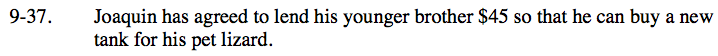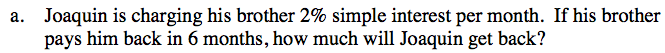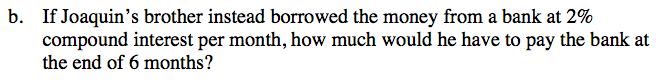### Home > CC3 > Chapter 9 > Lesson 9.1.3 > Problem9-37

9-37.
1. Joaquin has agreed to lend his younger brother $45 so that he can buy a new tank for his pet lizard. Homework Help ✎ 1. Joaquin is charging his brother 2% simple interest per month. If his brother pays him back in 6 months, how much will Joaquin get back? 2. If Joaquin’s brother instead borrowed the money from a bank at 2% compound interest per month, how much would he have to pay the bank at the end of 6 months?Using the equation for simple interest: Interest = Prt P = principal r = interest rate t = time Interest =$45(.02)(6 months)

\$50.40

Remember, the total money Joaquin gets back includes the principal and the interest.Use the equation for compound interest:

Total Amount = P(1 + r)n
P = principal
r = rate
n = number of times compounded

$45\left(1+.02\right)^6=45\cdot 1.02^6=50.68$